Symbolic execution has been a topic I have been meaning to jump into for a few months. Today we will look at how to apply symbolic execution to the Carnegie Melon Binary Bomb lab.

This entire writeup was performed in my Vagrant CTF VM EpicTreasure, which comes prepackaged with the tools necessary.

## Symbolic Execution?

For those unfamiliar with symbolic execution, I will present a summary of the mechanics as we proceed in the writeup. For further insight, I highly recommend checking out the MIT lecture on the subject (For real, if you haven’t seen symbolic execution before, this will appear to be black magic. A little background goes a long way).

In the reversing space, symbolic execution allows us as reverse engineers to find a given path from Address A to Address B given a certain input. This is accomplished by converting this reversing problem to a SAT problem where we can apply SMT solvers to determine a correct input.

## Problem at hand

Today we will tackle the CMU Binary Bomb, which presents a series of reverse engineering challenges in a single binary by which we have to determine the “password” for each level to proceed forward. We will begin with the second password as the first password was simply found with `strings` in the binary.

Firing up the binary in radare2, we will quickly reach the function in question.

We begin by analyzing everything with `aaa`.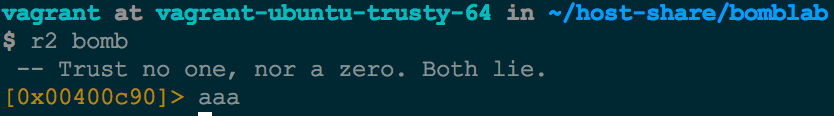A good first step is analyzing the identified functions with `afl`. We can `grep` for functions via the `~` operator on the command line to look for functions specific to each phase. We notice there is a function called `phase_2`.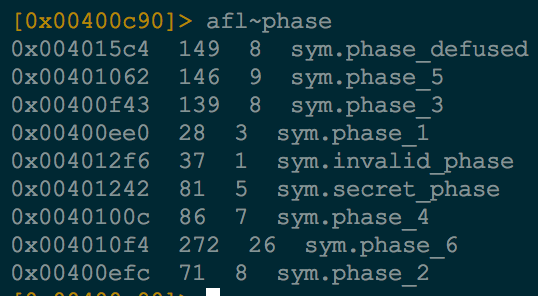We proceed to `seek` to our interesting function and then print the function contents with `pdf` for `[p]rint [d]issembly of [f]unction`.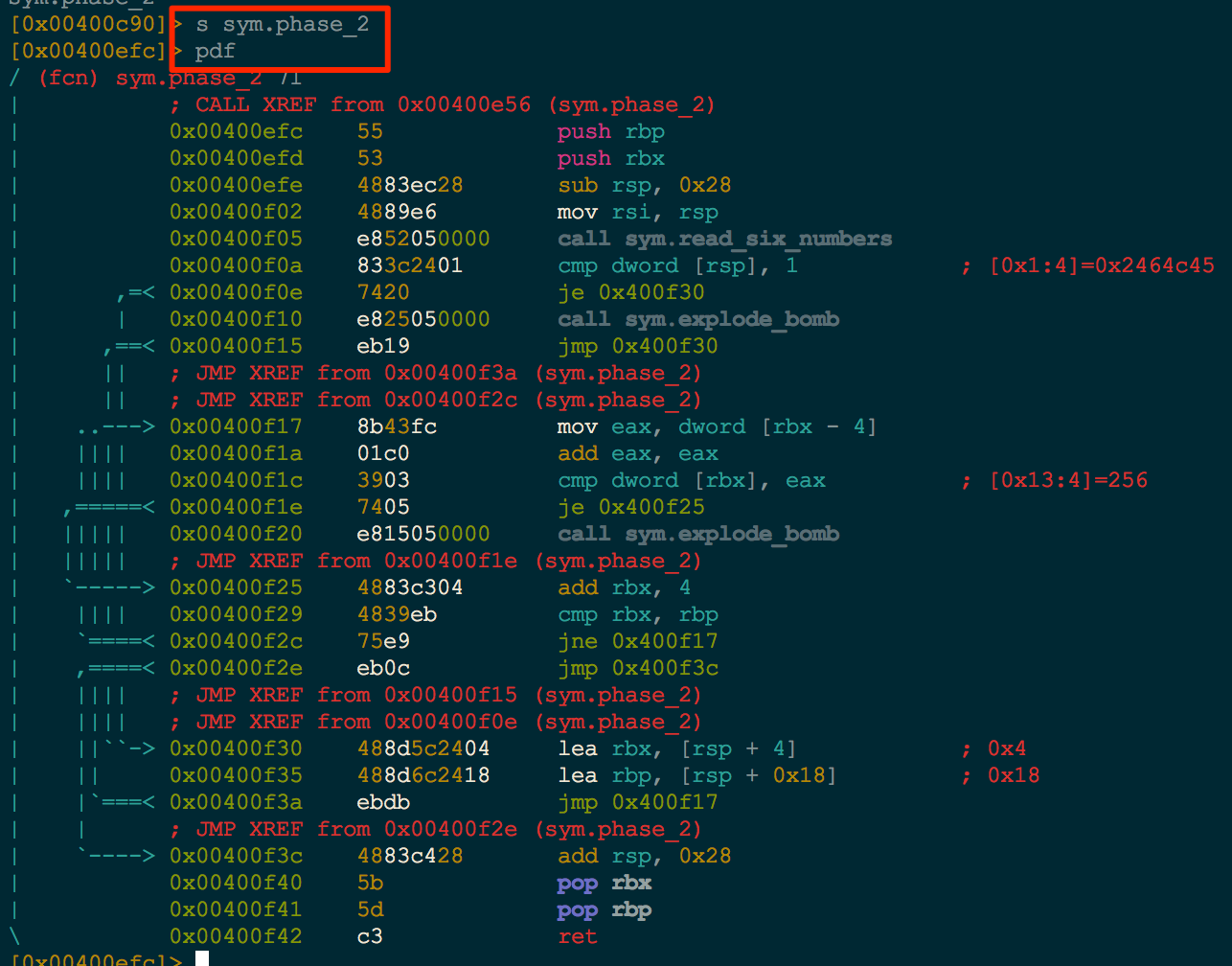This is a nice view for small functions, but large functions can be a bit difficult to traverse. With Radare’s Visual Mode `VV`, we can see an ascii conditional flow graph.In order for us to proceed with symbolic execution, the first step is to examine how the function is taking our data as input. The `read_six_numbers` function looks interesting. With radare in Visual Mode, simply pressing `ga` will jump to the `read_six_numbers` function (`g` for `goto symbol` and the `a` from the identified shortcut at `0x400f05` in the previous image).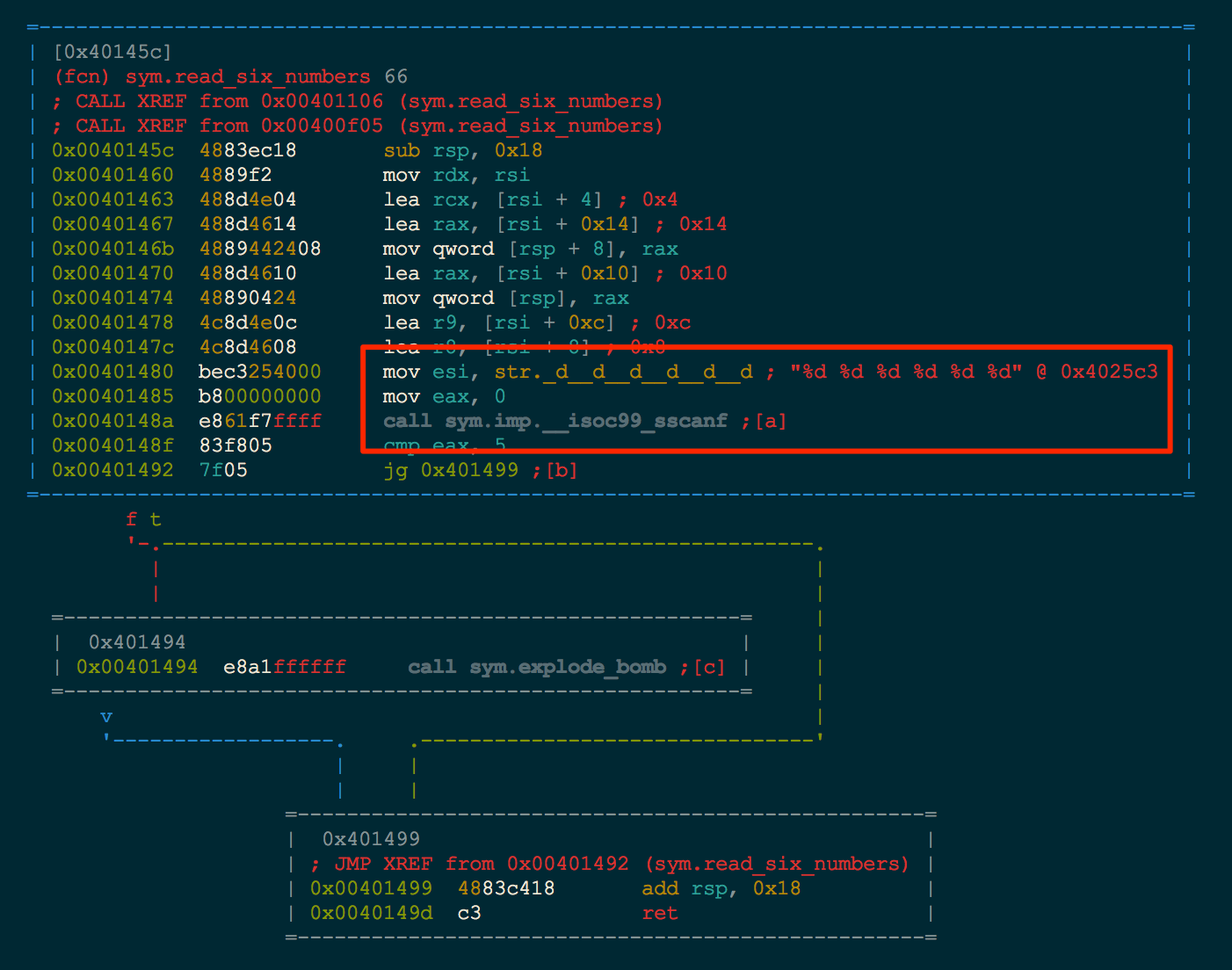The immediate function we recognize is the `scanf` reading values using the `%d %d %d %d %d %d` format string. This means we are looking for six 32-bit numbers as input to this function. To verify this assumption, we quickly drop into `pwndbg` and `break` just after the `read_six_numbers` function and give a sample input of `1 2 3 4 5 6` as input.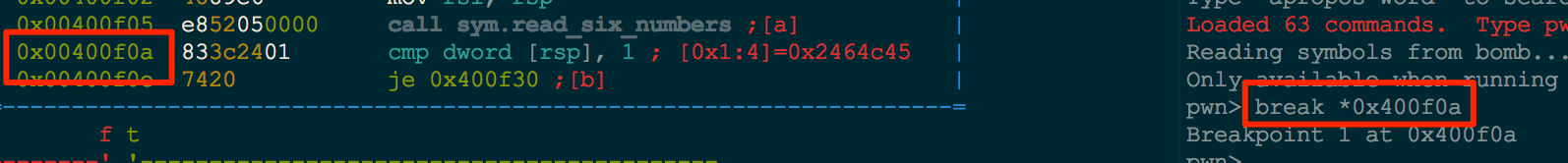Hitting the breakpoint, we examine what the stack looks like with `hexdump \$rsp`.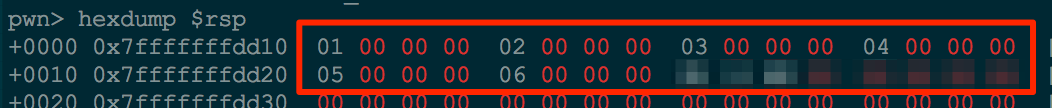Our assumption was correct; we do see six 32-bit values on the stack. We will create six 32-bit symbolic values once we begin our script.

The last piece of the puzzle that we need is to identify where we want to start execution, finish execution, and what part of execution we want to avoid. Let’s take a quick look at the overall function graph in radare (cycle through the modes with `p`). We can see that there are two locations `0x400f10` and `0x400f20` that both call the `explode_bomb` function.Taking a quick look at this function, we see that it prints out a few strings telling us that we have blown up the bomb and then an `exit`.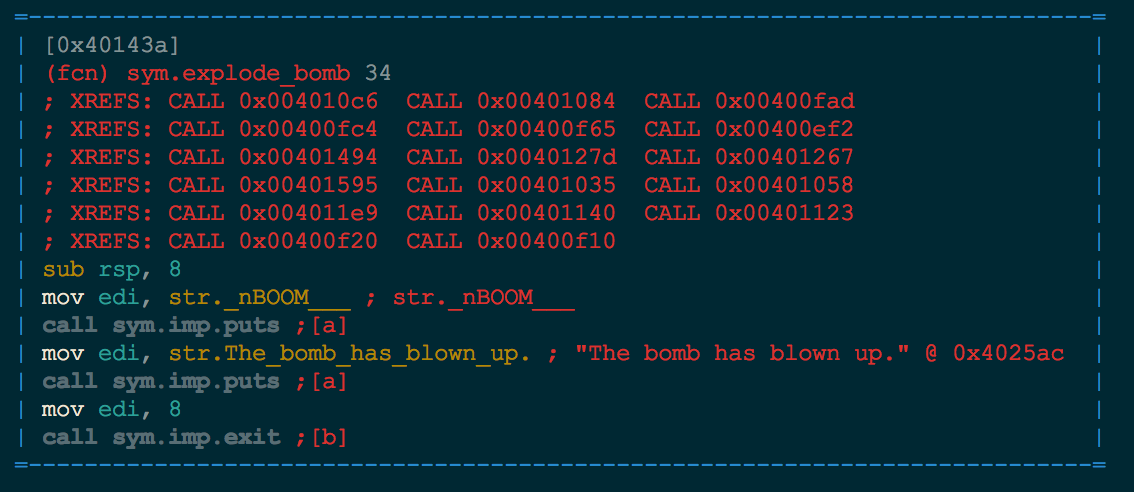This is obviously the part of execution that we want to avoid.

With that being found, we have our entire roadmap for symbolic execution. We have how input is received by the function. Assuming that if we return from the `phase_2` function and avoided the `bomb_explode` function we have succeeded, we know our start/finish/avoid functions. Our 10,000 ft roadmap looks something like this.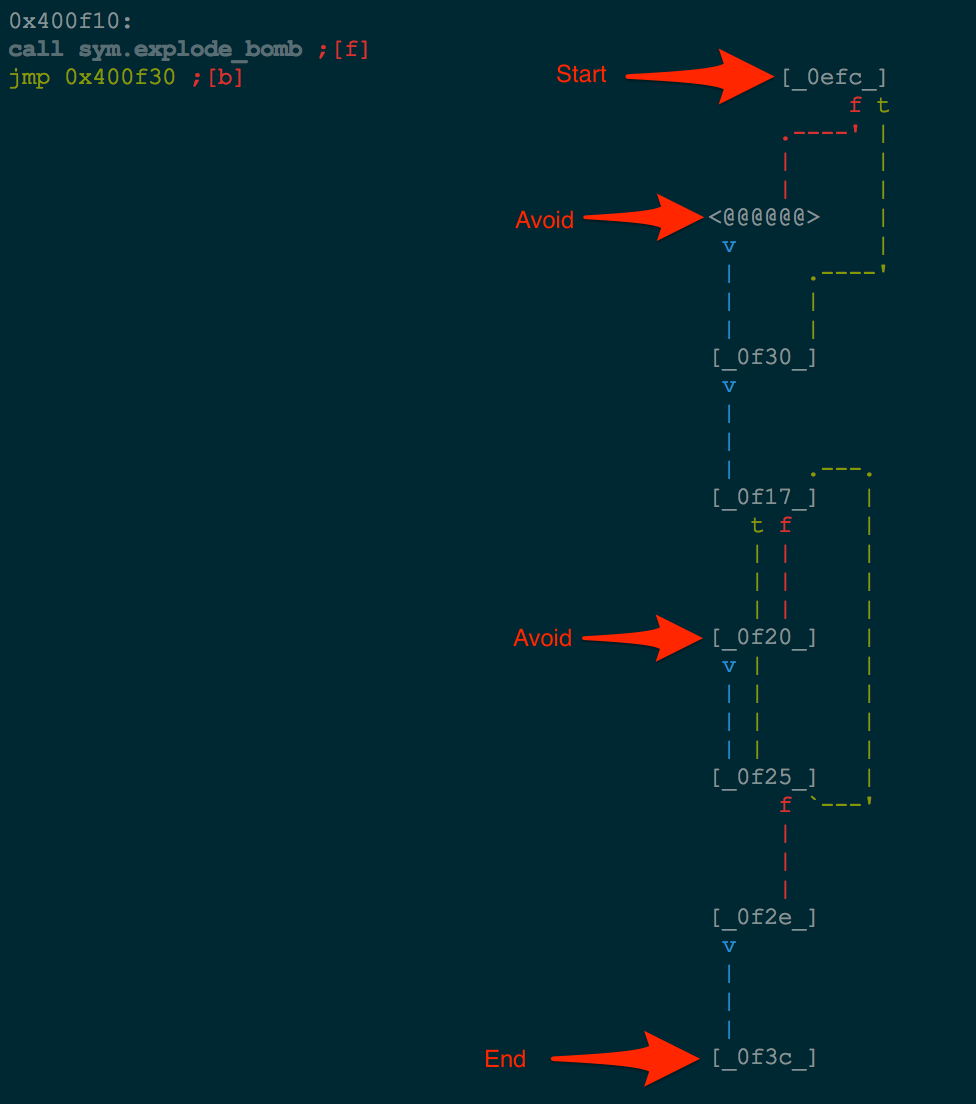## Symbolic Execution

With the pieces of the golden cup in hand, let’s begin constructing the script to solve this puzzle without actually solving the puzzle ourselves.

We will be using angr, a concolic execution engine developed by several researchers at the Computer Security Lab at UC Santa Barbara. This is the same engine that will be competing in the Finals for DARPA’s Cyber Grand Challenge. For more information on angr, check out their Docs.

We begin by handing our binary to angr.

``````proj = angr.Project('bomb', load_options={'auto_load_libs':False})
``````

We don’t want to run the entire binary to reach Phase 2 and simply want to start at Phase 2. This can be acheived with a `blank_state`. We begin execution at the instruction after the call to `read_six_numbers`.

``````state = proj.factory.blank_state(addr=0x400f0a)
``````

Because we are starting analysis in the middle of the binary and after the call to `scanf`, we have to construct the input ourselves. For this, we will create six 32-bit symbolic values and push those onto the stack, similar to what we recognized is the state of the binary after reading input from the example above.

``````for i in xrange(6):
state.stack_push(state.se.BVS('int{}'.format(i), 4*8))
``````

We use a `BVS` (Bit Vector Symbol) as the symbolic variable object. This is used internally by angr to create our equation that will be solved by angr’s SMT solver.

Now that our initial state is given, we simply create an `Explorer` object. We give this object where we start, where we want to finish, and what equations to avoid. The Explorer will then attempt to find a path following those constraints.

``````# ID from Radare
bomb_explode = 0x40143a
path = proj.factory.path(state=state)
ex = proj.surveyors.Explorer(start=path, find=(0x400f3c,),
avoid=(bomb_explode,), enable_veritesting=True)
ex.run()
``````

If a path has been found, we want to ask the SMT solver to attempt to solve the path equation it generated for what input will reach the end of our path. To accomplish this, we will pop values off of the stack at the end of the path and attempt to find valid integers at that time. Because this is a 64-bit binary, each value popped will be 64 bits. We will have do a little extraction of the two 32-bit values, but it will be painless.

``````if ex.found:
found = ex.found.state

for x in xrange(3):
curr_int = found.se.any_int(found.stack_pop())

# We are popping off 8 bytes at a time
# 0x0000000200000001

# This is just one way to extract the individual numbers from this popped value

``````

We grab the end state of a valid path from start to finish, avoiding the `bomb_explode` function. We simply pop values off of the stack and ask the SMT solver to give us a valid integer solution for those values. (`found.se.any_int(found.stack_pop())`) With this integer in hand, we extract the two 32-bit values to receive 2 of our 6 solutions. We proceed with this process 2 more times to result in the correct value.

## Final execution

The final solution execution is shown below.

## Final script

The final script is shown below.

``````## Binary found here: http://csapp.cs.cmu.edu/3e/bomb.tar

import angr, logging
from subprocess import Popen, PIPE
from itertools import product
import struct

def main():

logging.basicConfig()
logging.getLogger('angr.surveyors.explorer').setLevel(logging.DEBUG)

def nop(state):
return

bomb_explode = 0x40143a

# Start analysis at the phase_2 function after the sscanf

# Sscanf is looking for '%d %d %d %d %d %d' which ends up dropping 6 ints onto the stack
# We will create 6 symbolic values onto the stack to mimic this
for i in xrange(6):
state.stack_push(state.se.BVS('int{}'.format(i), 4*8))

# Attempt to find a path to the end of the phase_2 function while avoiding the bomb_explode
path = proj.factory.path(state=state)
ex = proj.surveyors.Explorer(start=path, find=(0x400f3c,),
avoid=(bomb_explode, 0x400f10, 0x400f20,),
enable_veritesting=True)
ex.run()
if ex.found:
found = ex.found.state

for x in xrange(3):
curr_int = found.se.any_int(found.stack_pop())

# We are popping off 8 bytes at a time
# 0x0000000200000001

# This is just one way to extract the individual numbers from this popped value
``git clone https://github.com/ctfhacker/ctf-writeups``